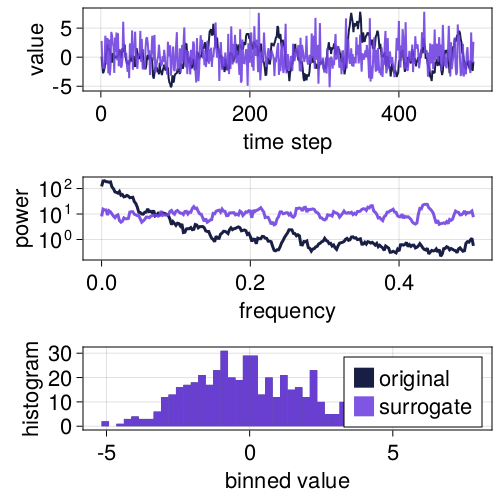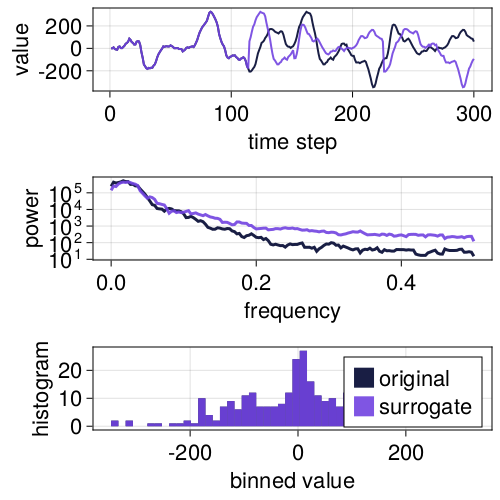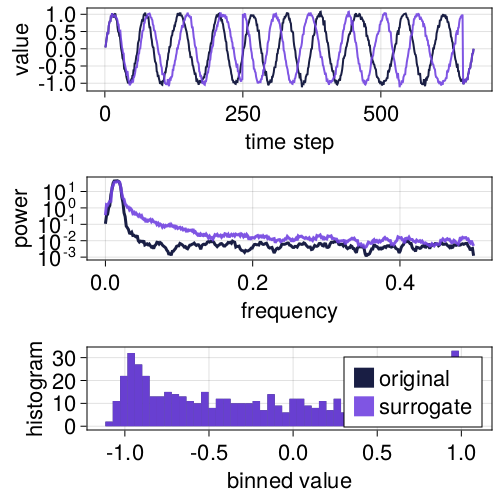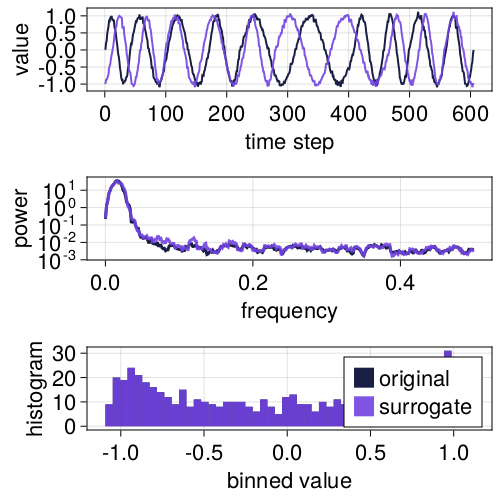# Shuffle-based

## Random shuffle (RS)

Randomly shuffled surrogates are simply permutations of the original time series.

Thus, they break any correlations in the signal.

using TimeseriesSurrogates, CairoMakie
x = AR1() # create a realization of a random AR(1) process
s = surrogate(x, RandomShuffle())
surroplot(x, s)## Block shuffle (BS)

Randomly shuffled surrogates are generated by dividing the original signal into blocks, then permuting those blocks. Block positions are randomized, and blocks at the end of the signal gets wrapped around to the start of the time series.

Thus, they keep short-term correlations within blocks, but destroy any long-term dynamical information in the signal.

using TimeseriesSurrogates, CairoMakie
x = NSAR2(n_steps = 300)
# We want to divide the signal into 8 blocks.
s = surrogate(x, BlockShuffle(8))
p = surroplot(x, s)## Cycle shuffle (CSS)

using TimeseriesSurrogates, CairoMakie
x = random_cycles()
s = surrogate(x, CycleShuffle())
p = surroplot(x, s)## Circular shift

using TimeseriesSurrogates, CairoMakie
x = random_cycles()
s = surrogate(x, CircShift(1:length(x)))
p = surroplot(x, s)